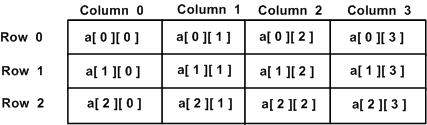Strategy

# Arrays in C/C++ – DZone Web Dev

C++ provides a data structure, the array, which stores a fixed-size, sequential collection of elements of the same type. They are used to store similar types of elements. (The data type must be the same for all elements.) They can be used to store collections of primitive data types, such as int, float, double, char, etc. of any particular type. To add to it, an array in C or C++ can store derived data types, such as structures, pointers etc.

All arrays consist of contiguous memory locations. The lowest address corresponds to the first element, and the highest address to the last element.

If you want to follow video tutorial, check it out below:

You may also like:
The Best of Java Collections [Tutorials].

## Array Declaration

To declare an array in C++, the programmer specifies the type of the elements and the number of elements in the array like:

This is called a single-dimension array. `arraySize` must be an integer constant greater than zero, and type can be any valid C++ data type. For example, to declare a 5-element array of type double, use this statement:

There are various ways in which we can declare an array. It can be done by specifying its type and size by initializing it or both.

1. Array declaration by specifying size
1. Array declaration by initializing elements
1. Array declaration by specifying size and initializing elements

Advantages of an Array in C/C++:

1. Random access of elements using array index.
2. Use less code, as it creates a single array of multiple elements.
3. Easy access to all the elements.
4. Traversal through the array becomes easy using a single loop.
5. Sorting becomes easy, as it can be accomplished by writing less code.

Disadvantages of an Array in C/C++:

1. Allows a fixed number of elements to be entered, which is decided at the time of declaration. Unlike a linked list, an array in C is not dynamic.
2. Insertion and deletion of elements can be costly since the elements are needed to be managed in accordance with new memory allocation.

Example of arrays:

Output:

Example of elements are stored at contiguous memory locations

Output:

## Multi-Dimensional Arrays

C++ allows multidimensional arrays. Here is the general form of a multidimensional array declaration −

For example, the following declaration creates a three dimensional 5 . 10 . 4 integer array −

### Two-Dimensional Arrays

The simplest form of the multidimensional array is the two-dimensional array. A two-dimensional array is, in essence, a list of one-dimensional arrays. To declare a two-dimensional integer array of size x,y, you would write something as follows:

`Type` can be any valid C++ data type, and `arrayName` will be a valid C++ identifier.

A two-dimensional array can be thought of as a table, which will have x number of rows and y number of columns. A two-dimensional array a, which contains three rows and four columns can be shown as follows:Every element in an array a is identified by an element name of the form a[ i ][ j ], where a is the name of the array, and i and j are the subscripts that uniquely identify each element in a.

#### Initializing Two-Dimensional Arrays

Multi-dimensioned arrays may be initialized by specifying bracketed values for each row. The following is an array with 3 rows, where each row has 4 columns.

The nested braces, which indicate the intended row, are optional. The following initialization is equivalent to previous example −

#### Accessing Two-Dimensional Array Elements

An element in a two-dimensional array is accessed by using subscripts (i.e., row index and column index of the array). For example:

The above statement will take the 4th element from the 3rd row of the array. You can verify this in the above diagram.

Output:

As explained above, you can have arrays with any number of dimensions, although it is likely that most of the arrays you create will be of one or two dimensions.

## Pointer to an Array

It is most likely that you would not understand this chapter until you have a decent understanding of pointers.

So, assuming you have bit understanding on pointers in C++, let us start: An array name is a constant pointer to the first element of the array. Therefore, in the declaration:

`balance` is a pointer to `&balance`, which is the address of the first element of the array balance. Thus, the following program fragment assigns `p` the address of the first element of `balance`:

It is legal to use array names as constant pointers, and vice versa. Therefore, `*(balance + 4)` is a legitimate way of accessing the data at `balance`.

Once you store the address of first element in `p`, you can access array elements using `*p`,  `*(p+1)`, `*(p+2)`, and so on. Below is the example to show all the concepts discussed above:

Output:

In the above example, `p` is a pointer to `double`, which means it can store an address of a variable of double type. Once we have address in p, then *p will give us value available at the address stored in p, as we have shown in the above example.